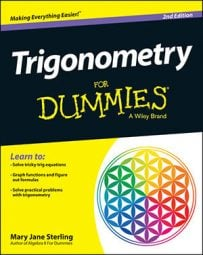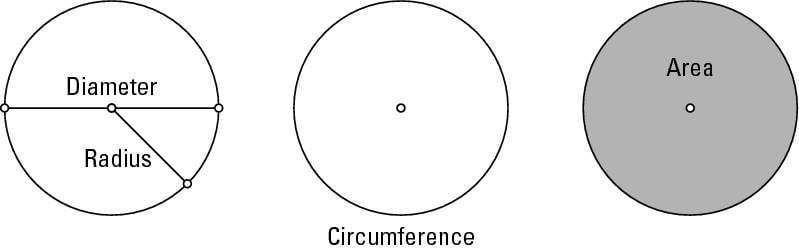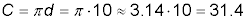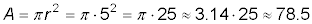##### Trigonometry For DummiesA circle is a geometric figure that needs only two parts to identify it and classify it: its center (or middle) and its radius (the distance from the center to any point on the circle). After you've chosen a point to be the center of a circle and know how far that point is from all the points that lie on the circle, you can draw a fairly decent picture.

With the measure of the radius, you can tell a lot about the circle: its diameter (the distance from one side to the other, passing through the center), its circumference (how far around it is), and its area (how many square inches, feet, yards, meters — what have you — fit into it).Ancient mathematicians figured out that the circumference of a circle is always a little more than three times the diameter of a circle. Since then, they narrowed that “little more than three times” to a value called pi (pronounced “pie”), designated by the Greek letter π.

The decimal value of π isn't exact — it goes on forever and ever, but most of the time, people refer to it as being approximately 3.14 or 22/7, whichever form works best in specific computations.

The formula for figuring out the circumference of a circle is tied to π and the diameter:

Circumference of a circle: C = πd = 2πr
The d represents the measure of the diameter, and r represents the measure of the radius. The diameter is always twice the radius, so either form of the equation works.

Similarly, the formula for the area of a circle is tied to π and the radius:

Area of a circle: A = πr2
This formula reads, “Area equals pi are squared.”

Find the radius, circumference, and area of a circle if its diameter is equal to 10 feet in length.

If the diameter (d) is equal to 10, you write this value as d = 10. The radius is half the diameter, so the radius is 5 feet, or r = 5. You can find the circumference by using the formulaSo, the circumference is about 31.5 feet around. You find the area by using the formulaso the area is about 78.5 square feet.